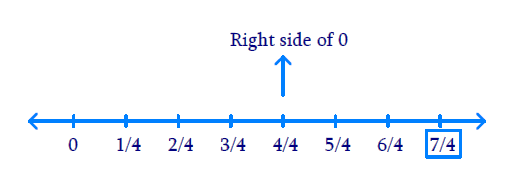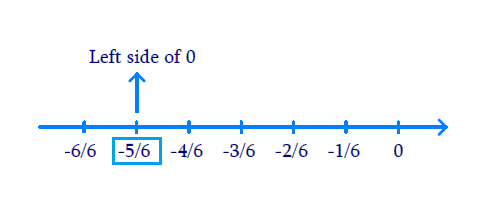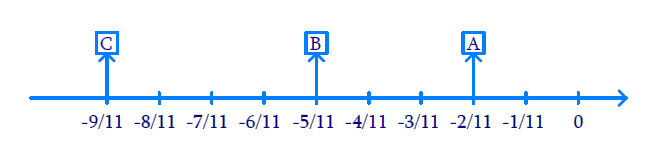# Excercise 1.2 Rational Numbers- NCERT Solutions Class 8

## Chapter 1 Ex.1.2 Question 1

Represent these numbers on the number line.

(i) \begin{align}\;\frac{7}{4}\end{align}

(ii) \begin{align}\;\frac{{ - 5}}{6}\end{align}

### Solution

Reasoning:

The positive numbers are on the right of $$0$$ and negative numbers are represented on the left of $$0$$ in the number line. The denominator of the rational number indicates the number of equal parts into which the first unit has to be divided whereas the numerator indicates as to how many of these parts are to be taken into consideration.

Steps:

\begin{align}\frac{7}{4}\end{align} The first unit has to be divided by $$4$$ parts. We make $$7$$ markings of distance

\begin{align}\frac{1}{4}\end{align} Each on the right of $$0$$ and starting from $$0.$$ The seventh marking represents \begin{align}\frac{7}{4}\end{align}(i) \begin{align}\frac{{ - 5}}{6}\end{align} The first unit has to be divided into $$5$$ parts. We make $$5$$ markings of distance \begin{align}\frac{1}{6}\end{align} each on the left of $$0$$ and starting from $$0.$$

The fifth marking represents \begin{align}\frac{5}{6}\end{align} left side of $$0.$$## Chapter 1 Ex.1.2 Question 2

Represent \begin{align}\frac{{ - 2}}{{11}},\;\;\frac{{ - 5}}{{11}},\;\;\frac{{ - 9}}{{11}}\end{align}  on the number line.

### Solution

Reasoning:

The negative numbers are represented on the left of $$0$$ in the number line. The denominator of the rational number indicates the number of equal parts into which the first unit has to be divided whereas the numerator indicates as to how many of these parts are to be taken into consideration.

Steps:

We make $$9$$ marking of distance \begin{align}\frac{1}{{11}}\end{align} each on the left of $$0$$ and starting from $$0.$$

The second marking represent \begin{align}\frac{{ - 2}}{{11}}\end{align} which is represented as $$A$$ in the line.

The fifth marking represent \begin{align}\frac{{ - 5}}{{11}}\end{align} representations $$B$$ in the line.

The ninth marking represent \begin{align}\frac{{ - 9}}{{11}}\end{align} represented as $$C$$ in the number line.## Chapter 1 Ex.1.2 Question 3

Write five rational numbers which are smaller than $$2.$$

### Solution

What is known?

Rational numbers.

What is unknown?

The rational numbers between given rational numbers.

Reasoning:

We can write infinitely many rational numbers lesser than number $$2.$$

Steps:

Five rational numbers which are smaller than $$2$$ are \begin{align}1,\;\;\frac{1}{2},\;\;0,\;\; - 1,\;\;\frac{{ - 1}}{2}\end{align}

## Chapter 1 Ex.1.2 Question 4

Find ten rational numbers between \begin{align}\frac{{ - 2}}{5}\end{align} and \begin{align}\frac{1}{2}\end{align}

### Solution

What is known?

Rational numbers.

What is unknown?

The rational numbers between given rational numbers.

Reasoning:

We can find infinitely many rational numbers between any two given rational numbers by taking the mean of the two rational numbers.

Another method: We can make the denominator same for the two given rational numbers.

Steps:

\begin{align}\frac{{ - 2}}{5} = \frac{{ - 2 \times 2}}{{5 \times 2}} = \frac{{ - 4}}{{10}}\end{align}  [multiplying both numerator and denominator by $$2$$]

\begin{align}\frac{1}{2} = \frac{{1 \times 5}}{{2 \times 5}} = \frac{5}{{10}}\end{align} [multiplying both numerator and denominator by $$5$$]

[Multiplying both numerators and denominators by the same number]

Now, there are $$9$$ rational numbers between \begin{align}\frac{{ - 4}}{{10}}\end{align} and \begin{align}\frac{5}{{10}}\end{align}, but we need $$10$$ numbers. So, we should again multiply both numerator and denominator by $$2$$ in the two rational numbers \begin{align}\frac{{ - 4}}{{10}}\end{align} and \begin{align}\frac{5}{{10}}\end{align}

\begin{align}\frac{{ - 4 \times 2}}{{10 \times 2}} = \frac{{ - 8}}{{20}}\end{align}and \begin{align}\frac{5}{{10}} \times \frac{2}{2} = \frac{{10}}{{20}}\end{align}

The ten rational numbers between \begin{align}\frac{{ - 2}}{5}\end{align} and \begin{align}\frac{1}{2}\end{align} which can be taken as.

\begin{align}&\frac{- 7}{20},\;\;\frac{- 6}{20},\;\;\frac{- 5}{20}, \;\;\frac{- 4}{20},\;\;\frac{- 3}{20},\;\; \\ & \frac{- 2}{20},\;\;\frac{1}{20},\;\;0,\;\;\frac{1}{20} \;\; \text{and} \;\; \frac{2}{20}\end{align}

[There are many more such rational numbers.]

## Chapter 1 Ex.1.2 Question 5

Find five rational numbers between,

(i) \begin{align}\;\frac{2}{3}\end{align} and \begin{align}\frac{4}{5}\end{align}

(ii)\begin{align}\;\frac{{ - 3}}{2}\end{align} and \begin{align}\frac{5}{3}\end{align}

(iii) \begin{align}\frac{1}{4}\end{align} and \begin{align}\frac{1}{2}\end{align}

### Solution

What is known?

Rational numbers.

What is unknown?

The rational numbers between given rational numbers.

Reasoning:

We can find infinitely many rational numbers between any two given rational numbers by taking the mean of the two rational numbers. Another method: We can make the denominator same for the two given rational numbers.

Steps:

(i)   \begin{align}\frac{2}{3}\end{align} and \begin{align}\frac{4}{5}\end{align}

\begin{align}\frac{2}{3} = \frac{{2 \times 20}}{{3 \times 20}} = \frac{{40}}{{60}}\end{align} [multiplying both numerator and denominator by \begin{align}20\end{align}]

\begin{align}\frac{4}{5} = \frac{{4 \times 12}}{{5 \times 12}} = \frac{{48}}{{60}}\end{align} [multiplying both numerator and denominator by \begin{align}12\end{align}]

The five rational numbers between \begin{align}\frac{2}{3}\end{align} and \begin{align}\frac{4}{5}\end{align} that can be taken are:

\begin{align}\frac{{41}}{{60}},\;\;\frac{{42}}{{60}},\;\;\frac{{43}}{{60}},\;\;\frac{{44}}{{60}},\;\;\frac{{45}}{{60}}\end{align}

(ii)   \begin{align}\frac{{ - 3}}{2}\end{align} and \begin{align}\frac{5}{3}\end{align}

\begin{align}\frac{{ - 3}}{2} = \frac{{ - 3 \times 3}}{{2 \times 3}} = \frac{{ - 9}}{6}\end{align} [multiplying both numerator and denominator by \begin{align}3\end{align}]

\begin{align}\frac{5}{3} = \frac{{5 \times 2}}{{2 \times 3}} = \frac{{10}}{6}\end{align} [multiplying both numerator denominator by \begin{align}2\end{align}]

\begin{align}\therefore\end{align} The five rational numbers between \begin{align}\frac{{ - 3}}{2}\end{align} and \begin{align}\frac{5}{3}\end{align} that can be taken are:

\begin{align} - \frac{8}{6},\;\; - \frac{7}{6},\;\;-1,\;\;\frac{5}{6},\;\;\frac{4}{6}\end{align}

[There can be more such rational numbers]

(iii)   \begin{align}\frac{1}{4}\end{align} and \begin{align}\frac{1}{2}\end{align}

\begin{align}\frac{1}{4} = \frac{{1 \times 8}}{{4 \times 8}} = \frac{8}{{32}}\end{align} [multiplying both numerator and denominator by \begin{align}8\end{align}]

\begin{align}\frac{1}{2} = \frac{{1 \times 16}}{{2 \times 16}} = \frac{{16}}{{32}}\end{align} [multiplying both numerator and denominator by \begin{align}16\end{align}]

Thus, five rational numbers between \begin{align}\frac{1}{2}\end{align} and \begin{align}\frac{1}{4}\end{align} that can be taken are:

\begin{align}\frac{9}{{32}},\;\;\frac{{10}}{{32}},\;\;\frac{{11}}{{32}},\;\;\frac{{12}}{{32}},\;\;\frac{{13}}{{32}}\end{align}

## Chapter 1 Ex.1.2 Question 6

Write five rational numbers greater than $$–2.$$

### Solution

What is known?

Rational numbers.

What is unknown?

The rational numbers between given rational numbers.

Reasoning:

We can find infinitely many rational numbers between any two given rational numbers by taking the mean of the two rational numbers. Another method: We can make the denominator same for the two given rational numbers.

Steps:

Some of the five rational numbers greater than $$– 2$$ are \begin{align}- 1,\;\;0,\;\;\frac{1}{2},\;\;\frac{1}{3}, \end{align} and \begin{align}\frac{1}{4} \end{align}

[There can be more such rational numbers]

## Chapter 1 Ex.1.2 Question 7

Find the rational numbers between \begin{align}\frac{3}{5}\end{align} and \begin{align}\frac{3}{4}\end{align}

### Solution

What is known?

Rational numbers.

What is unknown?

The rational numbers between given rational numbers.

Reasoning:

We can find infinitely many rational numbers between any two given rational numbers by taking the mean of the two rational numbers.

Another method: We can make the denominator same for the two given rational numbers.

Steps:

\begin{align}\frac{3}{5} = \frac{{3 \times 32}}{{5 \times 32}} = \frac{{96}}{{160}}\end{align} [multiplying both numerator and denominator by $$32$$]

\begin{align}\frac{3}{4} = \frac{{3 \times 40}}{{4 \times 40}} = \frac{{120}}{{160}} \end{align} [multiplying both numerator and denominator by $$40$$]

Thus, ten rational numbers between \begin{align}\frac{3}{5}\end{align} and \begin{align}\frac{3}{4}\end{align} that can be written are:

\begin{align} &\frac{97}{160},\;\;\frac{98}{160},\;\;\frac{99}{160},\;\;\frac{100}{160},\;\;\frac{101}{160},\;\; \\ & \frac{102}{160},\;\;\frac{103}{160},\;\;\frac{104}{160},\;\;\frac{105}{160},\;\;\frac{106}{160}\end{align}

[There can be many more such rational numbers]

Instant doubt clearing with Cuemath Advanced Math Program